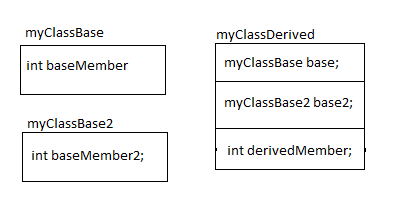# Multiple Inheritance (Multiple Base Classes)

Consider these classes:
```class myClassBase
{
public:
int baseMember;
myClassBase() { baseMember = 0; }
int baseMethod(int x) { return baseMember + x; }
};
class myClassBase2
{
public:
int baseMember2;
myClassBase2() { baseMember2 = 0; }
int baseMethod2(int x) { return baseMember2 + x; }
};
class myClassDerived : public myClassBase, public myClassBase2
{
public:
int derivedMember;
myClassDerived() { derivedMember = 0; }
int derivedMethod(int x) { return derivedMember + x; }
};
```

The equivalent C code will be -
```struct myClassBase
{
int baseMember;
};
struct myClassBase2
{
int baseMember2;
};
struct myClassDerived
{
struct myClassBase base;
struct myClassBase2 base2;
int derivedMember;
};
void myClassBase_ctor(struct myClassBase *this)
{
this->baseMember = 0;
}
int myClassBase_baseMethod(struct myClassBase *this, int x)
{
return this->baseMember + x;
}
void myClassBase2_ctor(struct myClassBase2 *this)
{
this->baseMember2 = 0;
}
int myClassBase2_baseMethod(struct myClassBase2 *this, int x)
{
return this->baseMember2 + x;
}
void myClassDerived_ctor(struct myClassDerived *this)
{
myClassBase_ctor((struct myClassBase*)this);
void *ptr = (void*)this + offsetof(struct myClassDerived, base2);
myClassBase2_ctor((struct myClassBase2*)ptr);
this->derivedMember = 0;
}
int myClassDerived_derivedMethod(struct myClassDerived *this, int x)
{
return this->derivedMember + x;
}
```

Here is diagram describing the all three class relations:Points to understand:
• The derived class object contains objects of base classes embedded into this. This is in the same sequence as written in C++ code. The object of derived class will first have all the objects of base classes then its own data member. But this may depend on compiler implementation. The example given here is based on g++ compiler.
• The constructor of derived class calls the constructor of all base classes. While calling the constructor of a base class, the derived class passes the pointers to base type. The derived class constructor has the information of offset of the base class object inside the derived class object. It sets the pointer appropriately. In the example given above, the object of myClassBase starts at zero offset, so it uses the same pointer as that of myClassDerived to call the constructor of myClassBase. And the object of myClassBase2 starts inside the object of the derived class object. So it calculates the offset using offsetof macro and then uses the pointer at that offset for calling the constructor of myClassBase2.
• Whenever a derived class object is casted into one of the base class object, then the pointer is set to an offset in the object of the derived class from where the base class object is located.

Now you can understand how this code works-
```myClassDerived *derived = new myClassDerived;
void *ptr = derived;
myClassDerived * derived2 = (myClassDerived *)ptr;
myClassBase *base1 = (myClassBase *)ptr;
```

Question: What is the issue with this code?
```myClassBase2 *base2 = (myClassBase2 *)ptr;
```

Here the myClassBase2 object is casted from a void pointer. The compiler has no idea what is the offset of the myClassBase2 in the given object. In this case the compiler points to the first byte which is wrong in this case. If you will cast the myClassDerived* to myClassBase2* then the compiler knows the offset of myClassBase2 in the myClassDerived. The compiler knows it from the inheritance structure of the derived class and hence it will generate code to point to correct offset in the derived class object.

Do you collaborate using whiteboard? Please try Lekh Board - An Intelligent Collaborate Whiteboard App